Friction / Frictional ForceFriction 摩 擦力 mó cā lì is a force 力 lì that opposes (goes against) motion. Friction 摩擦力 mó cā lì occurs between two surfaces 表面 biǎo miàn that are in contact 接 触 jiē chù.

If an object 物体 wù tǐ lying on a surface is exerted (given) a push tuī (
force 力 lì) of F1 Newtons and the frictional force 力 lì that exists between the object 物 体 wù tǐ and the surface 表 面 biǎo miàn is F2, then the resultant force, FR is as follows.

Resultant force,
FR F1- F2

According to Newton's 2nd Law of Motion,
FR= ma
where
m =
mass 质 量 zhì liàng
a =
acceleration 加速度 jiā sù dù

Therefore,
FR F1- F2
or
ma = F1- F2

The object would then move at an
acceleration 加速度 jiā sù dù of
a = (
F1- F2) / m
if there is a positive
resultant force..

If this knol is helpful, please Like and Share it. Thanks.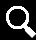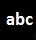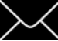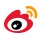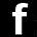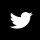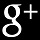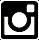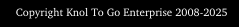Daya Geseran

Daya geseran merupakan daya yang menentang gerakan. Geseran wujud di antara dua permukaan yang bersentuh. Sekiranya, satu objek dikenakan daya tolakan bersamaan F1 Newton dan daya geseran di antara objek dan permukaan ialah F2 ,  maka daya paduan FR ialah

Daya paduan, FR F1- F2

Menurut Hukum Gerakan Newton Kedua
FR= ma
di mana
m =
jisim
a = pecutan

Yakni,
FR F1- F2
or
ma = F1- F2

Objek akan bergerak pada pecutan
a = (
F1- F2) / m
sekiranya terdapat
daya paduan yang positif.

Sekira knol ini memanfaatkan anda, sila tekan Like dan Kongsikannya. Terima kasih.